Electron. J. Diff. Equ., Vol. 2015 (2015), No. 52, pp. 1-12.

### On a sharp condition for the existence of weak solutions to the Dirichlet problem for degenerate nonlinear elliptic equations with power weights and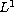-data Alexander A. Kovalevsky, Francesco Nicolosi

Abstract:
In this article, we establish a sharp condition for the existence of weak solutions to the Dirichlet problem for degenerate nonlinear elliptic second-order equations with-data in a bounded open set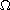of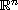with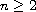. We assume thatcontains the origin and assume that the growth and coercivity conditions on coefficients of the equations involve the weighted function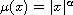, where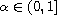, and a parameter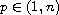. We prove that if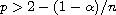, then the Dirichlet problem has weak solutions for every-right-hand side. On the other hand, we find that if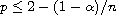, then there exists an-datum such that the corresponding Dirichlet problem does not have weak solutions.

Submitted August 5, 2014. Published February 25, 2015.
Math Subject Classifications: 35J25, 35J60, 35J70, 35R05.
Key Words: Degenerate nonlinear elliptic second-order equation;-data; power weights; Dirichlet problem; weak solution; existence and nonexistence of weak solutions.

Show me the PDF file (256 KB), TEX file, and other files for this article.Alexander A. Kovalevsky Department of Equations of Mathematical Physics Krasovsky Institute of Mathematics and Mechanics Ural Branch of Russian Academy of Sciences Ekaterinburg, Russia email: alexkvl71@mail.ru Francesco Nicolosi Department of Mathematics and Informatics University of Catania Catania, Italy email: fnicolosi@dmi.unict.it